Function Repository Resource:

# CalkinWilf

Get the position of a fraction or vice-versa within the Calkin–Wilf sequence

Contributed by: Ed Pegg Jr
 ResourceFunction["CalkinWilf"][n] returns the fraction at integer position n in the Calkin–Wilf sequence. ResourceFunction["CalkinWilf"][frac] returns the integer position of fraction frac in the Calkin–Wilf sequence. ResourceFunction["CalkinWilf"][numer,denom] returns the integer position of fraction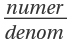in the Calkin–Wilf sequence.

## Details

The Calkin–Wilf binary tree has nodes of type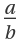with subnodes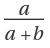and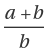.
The breadth-first traversal of the Calkin–Wilf tree gives the Calkin–Wilf sequence, with initial terms shown in the next cell.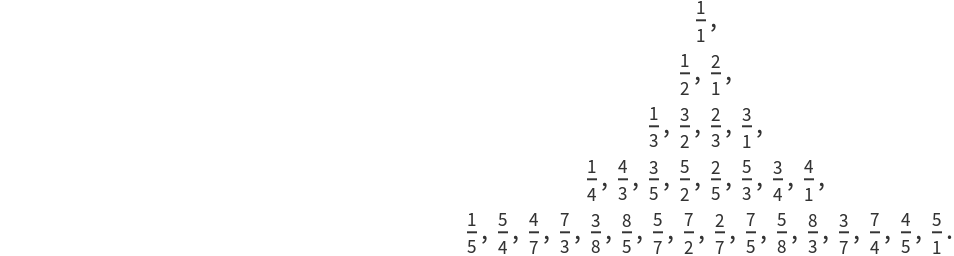In the positive Calkin–Wilf sequence, the denominator of term aj is the numerator of term aj+1. The series of numerators is known as the Fusc sequence.
In this function implementation, f=0 and f[-n]=-f[n], which may be considered nonstandard.
A bit-reversal permutation of the Calkin–Wilf tree gives the Stern–Brocot tree.
The binary length of the index for fraction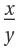equals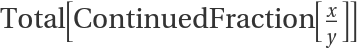. This can lead to unexpected results. For example,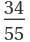has index 426, but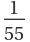has index 18014398509481984.

## Examples

### Basic Examples (2)

Find the position of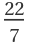in the Calkin–Wilf sequence:

 In:=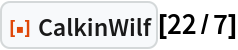Out=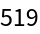Find the position ofin the Calkin–Wilf sequence with explicit numerator and denominator:

 In:=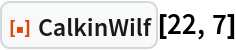Out=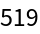Find the fraction at position 519 in the Calkin–Wilf sequence:

 In:=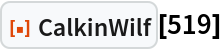Out=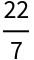Generate terms of the Farey sequence:

 In:=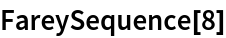Out=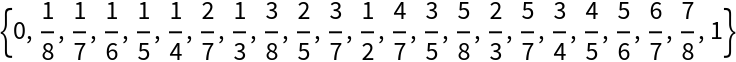Show their positions in the Calkin–Wilf sequence:

 In:=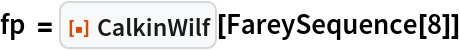Out=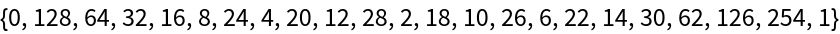Use the positions to generate fractions:

 In:=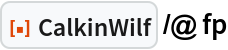Out=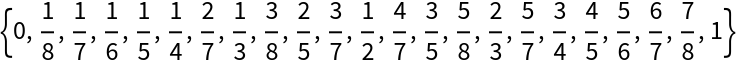### Scope (4)

Show the first 42 terms:

 In:=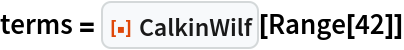Out=Get back the positions using explicit numerators and denominators:

 In:=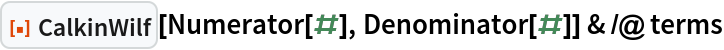Out=Get back the positions by forcing a denominator of 1 everywhere:

 In:=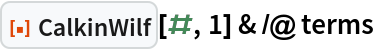Out=A two term form is needed for positions of integers since fractions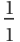,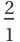,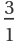,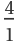are returned as 1, 2, 3, 4:

 In:=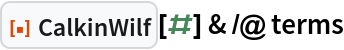Out=### Properties and Relations (7)

Negative values return negative fractions:

 In:=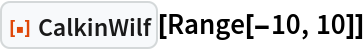Out=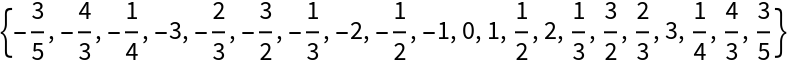Negative fractions correspond to negative positions:

 In:=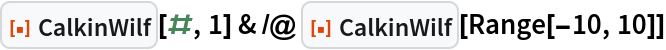Out=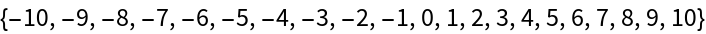Terms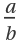and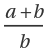are at positions k and 2k+1:

 In:=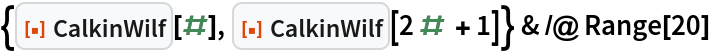Out=Level 5 of the Calkin–Wilf tree:

 In:=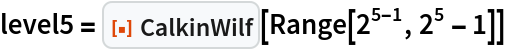Out=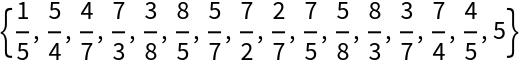Find the Total of the terms of the ContinuedFraction for level 5 of the Calkin–Wilf tree:

 In:=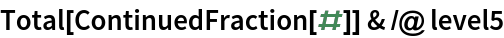Out=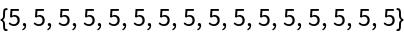For terms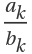on the same level in the Calkin–Wilf tree,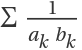=1:

 In:=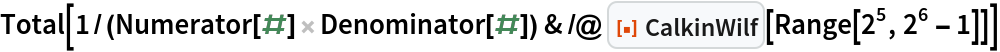Out=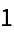The maximal numerator for level n of the Calkin–Wilf tree is Fibonacci[n+1]:

 In:=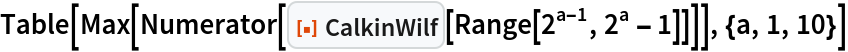Out=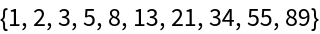An unreduced fraction gives the same value as the reduced fraction:

 In:=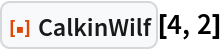Out=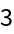In:=Out=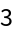Create a plot of the first 256 values:

 In:=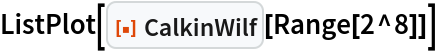Out=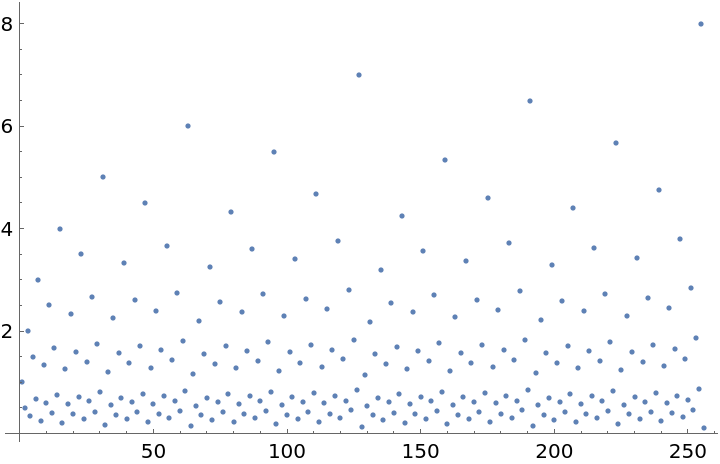Create a plot of indices for the Farey sequence fractions:

 In:=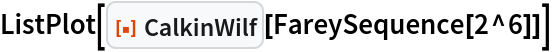Out=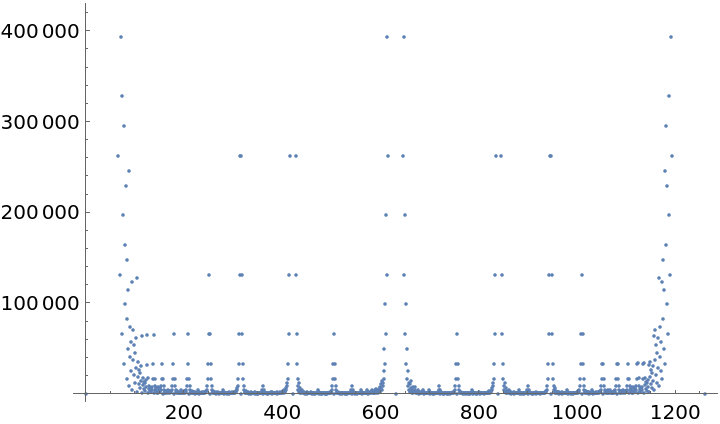### Possible Issues (3)

Fractions at positions 2a-1 are returned as a instead of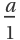:

 In:=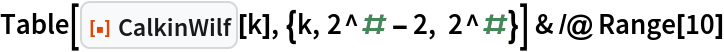Out=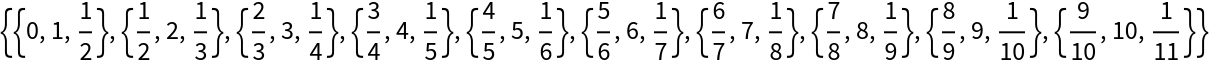CalkinWilf returns a Failure if its input is anything other than an rational:

 In:=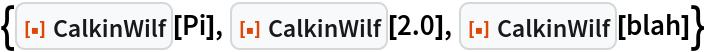Out=Fractions leading to an index with more than a million digits will be flagged as out of range:

 In:=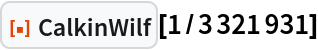Out=Fractions with a million digit index will be evaluated as expected:

 In:=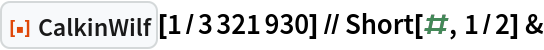Out=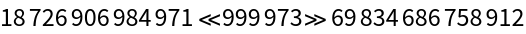### Neat Examples (2)

Show the Calkin–Wilf binary tree with nodes of typeand sub-nodes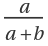and:

 In:=Out=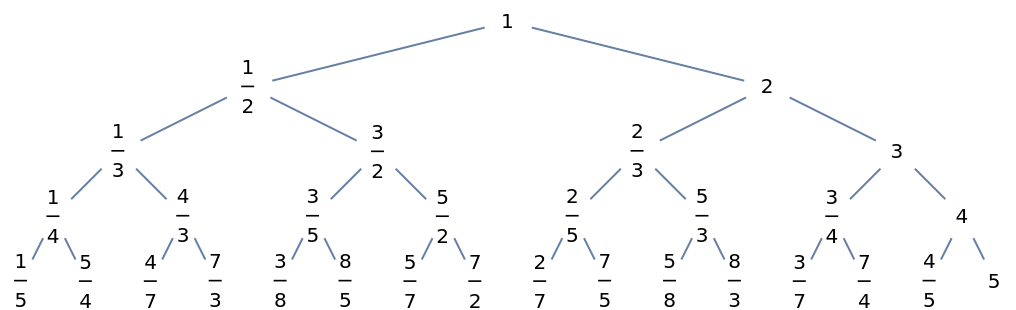List the denominators of the Calkin–Wilf sequence:

 In:=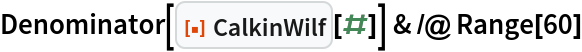Out=Prepend a 1 to get the numerators of the Calkin–Wilf sequence:

 In:=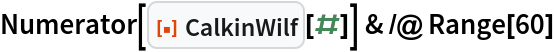Out=This is also known as the Fusc sequence:

 In:=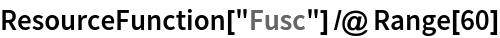Out=Plot the Fusc sequence:

 In:=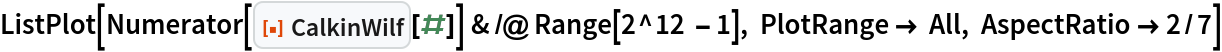Out=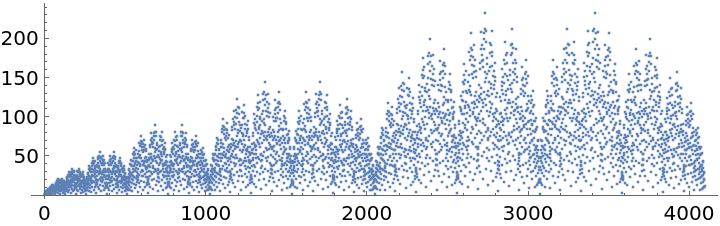## Version History

• 1.0.0 – 03 January 2023